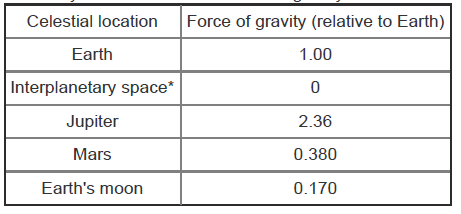# Problem: An object with a mass of 245 kg was weighed at each of the celestial locations given in the table. Rank these locations by the weight of the object at that location.a. Jupiterb. Earthc. Marsd. Earth's moone. Interplanetary spaceMass (mm) is defined as the amount of material in an object. The SI base unit of mass is the kilogram, kg. Weight (WW) is the force that the mass exerts due to gravity (gg). Weight is directly proportional to mass as shown by the equationW = m•gWeight is measured in force units such as newtons, N, or pounds, lb. Thus, gg has units of weight per mass. On Earth, gg is equal to 9.81 N/kg or 1.00 lb/ lb. Note that pounds can measure both weight and mass.Weight varies directly with the force of gravity exerted on the object and will be different at various locations in the solar system. Each planet exerts a certain gravitational force, and the weight of an object on that planet will vary directly with the magnitude of this force. Mass however is constant because it does not depend on gravity.The following table lists the force of gravity at various locations in the solar system relative to the force of gravity on Earth.*Refers to a position between planets where gravitational attraction is negligible.

###### FREE Expert Solution
98% (276 ratings)View Complete Written Solution
###### Problem Details

An object with a mass of 245 kg was weighed at each of the celestial locations given in the table.

Rank these locations by the weight of the object at that location.

a. Jupiter

b. Earth

c. Mars

d. Earth's moon

e. Interplanetary space

Mass (mm) is defined as the amount of material in an object. The SI base unit of mass is the kilogram, kg. Weight (WW) is the force that the mass exerts due to gravity (gg). Weight is directly proportional to mass as shown by the equation

W = m•g

Weight is measured in force units such as newtons, N, or pounds, lb. Thus, gg has units of weight per mass. On Earth, gg is equal to 9.81 N/kg or 1.00 lb/ lb. Note that pounds can measure both weight and mass.

Weight varies directly with the force of gravity exerted on the object and will be different at various locations in the solar system. Each planet exerts a certain gravitational force, and the weight of an object on that planet will vary directly with the magnitude of this force. Mass however is constant because it does not depend on gravity.

The following table lists the force of gravity at various locations in the solar system relative to the force of gravity on Earth.*Refers to a position between planets where gravitational attraction is negligible.

Frequently Asked Questions

What scientific concept do you need to know in order to solve this problem?

Our tutors have indicated that to solve this problem you will need to apply the Dimensional Analysis concept. You can view video lessons to learn Dimensional Analysis. Or if you need more Dimensional Analysis practice, you can also practice Dimensional Analysis practice problems.

What professor is this problem relevant for?

Based on our data, we think this problem is relevant for Professor Gines' class at UVU.